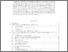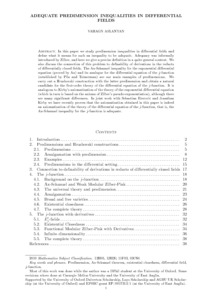# Adequate predimension inequalities in differential fields

Aslanyan, Vahagn (2022) Adequate predimension inequalities in differential fields. Annals of Pure and Applied Logic, 173 (1). ISSN 0168-0072Preview PDF (Adequate_predimension_inequalities_in_differential_fields) - Accepted Version Available under License Creative Commons Attribution Non-commercial No Derivatives. Download (707kB) | Preview

## Abstract

In this paper we study predimension inequalities in differential fields and define what it means for such an inequality to be adequate. Adequacy was informally introduced by Zilber, and here we give a precise definition in a quite general context. We also discuss the connection of this problem to definability of derivations in the reducts of differentially closed fields. The Ax-Schanuel inequality for the exponential differential equation (proved by Ax) and its analogue for the differential equation of the j-function (established by Pila and Tsimerman) are our main examples of predimensions. We carry out a Hrushovski construction with the latter predimension and obtain a natural candidate for the first-order theory of the differential equation of the j-function. It is analogous to Kirby's axiomatisation of the theory of the exponential differential equation (which in turn is based on the axioms of Zilber's pseudo-exponentiation), although there are many significant differences. In joint work with Sebastian Eterović and Jonathan Kirby we have recently proven that the axiomatisation obtained in this paper is indeed an axiomatisation of the theory of the differential equation of the j-function, that is, the Ax-Schanuel inequality for the j-function is adequate.

Item Type: Article Faculty of Science > School of Mathematics LivePure Connector 27 Nov 2021 01:48 22 Oct 2022 16:30 https://ueaeprints.uea.ac.uk/id/eprint/82386 10.1016/j.apal.2021.103030View Item